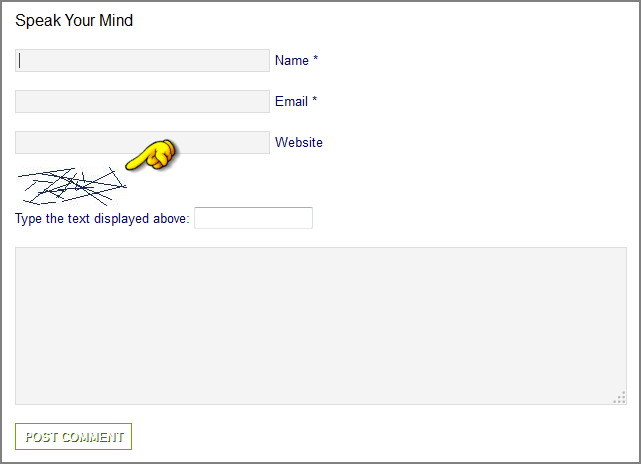# Making CAPTCHA Friendlier with Simple Number Tests or PHP Image Manipulation

Published on
59,564 Points
12,064 Views
15 Endorsements
Awarded

Things That Drive Us Nuts

Have you noticed the use of the reCaptcha feature at EE and other web sites?  It wants you to read and retype something that looks like this.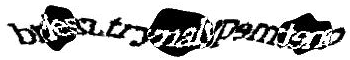Insanity!  It's not EE's fault - that's just the way reCaptcha works.  But it is a far cry from a good user experience.

Anti-Spam and Anti-'Bot Tools (Power to the People)

The term CAPTCHA is "sort of" an acronym.  It stands for Completely Automated Public Test (to tell) Computers and Humans Apart.  The theory is fairly simple.  Your server-side script gives the client a test that a human can pass easily but a computer cannot readily understand.  You can read more about the theory and implementations here, and in the Wikipedia.

A "honeypot" is a form element that should not be filled in when a human completes the form.  You can give a form input control a tempting name, like "email" and style the input with CSS to make it invisible on the browser.  If the form contains any data in the tempting input field, you can discard the request, since this would not have come from a human being.

```<?php // RAY_honeypot.php
error_reporting(E_ALL);

// DEMONSTRATE A HONEYPOT CAPTCHA TEST

// IF THE FORM HAS BEEN FILLED IN
if (!empty(\$_POST))
{
// IF THE HONEYPOT HAS BEEN FILLED IN
if (!empty(\$_POST['email'])) trigger_error("BE GONE, ATTACK BOT!", E_USER_ERROR);

// PROCESS THE REST OF THE FORM DATA HERE
var_dump(\$_POST);
}

// CREATE THE FORM
\$form = <<<EOD
<style type="text/css">
.honey {
display:none;
}
</style>
<form method="post">
<input name="email" class="honey" />
<input name="thing" />
<input type="submit" />
</form>
EOD;

echo \$form;```

One step up from an invisible CAPTCHA might be a checkbox that says, "Check this box to prove you're a human."  Not very deep, but arguably effective in a limited way.  And there is this from the endearing "A Word a Day" site.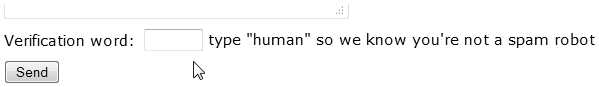Another simple design pattern is a form field that has a value filled in.  The web page asks the human to clear the field before submitting the form.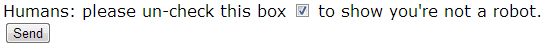Visually Based CAPTCHA Test

To reduce the risk of automated registration, the Craftsy web site uses a simple visual CAPTCHA.  The client is asked what animal is shown.  Craftsy may find the 1:4 ratio of possibilities acceptable; statistically speaking, an attack 'bot could be right about the animal 25% of the time.  If Craftsy couples its CAPTCHA with some kind of email verification this is probably acceptable protection.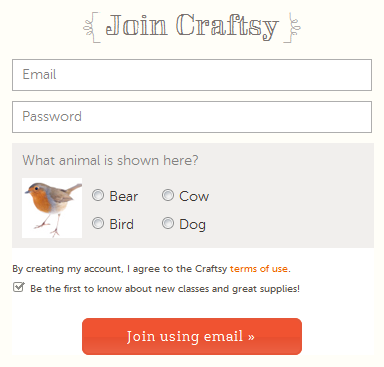At a slightly higher level, when there is common knowledge in a community, you might ask the client to enter the name of, for example, the school mascot.  The server-side verification for these tests is very simple, usually only a single if() statement.

A CAPTCHA Test with Simple Arithmetic

You can copy this script, put it on your server and run it to see the effect.  The script chooses two numbers at random, then chooses among several possible arithmetic operations to produce a CAPTCHA test that writes out an English-language simple math problem.  The client experience in this structure is very similar to the CAPTCHA test on the comment feature of the PHP.net web site.  It is easy to implement and easy for the client to use, but for a 'bot to readily defeat it, there would be a lot of programming required.  The web site would use the getQuestion() method in the HTML form script, and would use the testAnswer() method in the action= script. Give it a try, and if it's good enough for your work, enjoy it.  And if you feel you need greater obscurity, read on below for the image-based CAPTCHA tests.

```<?php // RAY_captcha_class.php
error_reporting(E_ALL);

// DEPENDS ON THE PHP SESSION
session_start();
echo '<pre>';

{
// NULL CONSTRUCTOR
public function __construct() { }

// RETURN A CAPTCHA QUESTION IN THE FORM OF A STRING
public function getQuestion()
{
// NUMBER NAMES CONVENIENTLY INDEXED BY VALUES
\$nums = array
( 'Zero'
, 'One'
, 'Two'
, 'Three'
, 'Four'
, 'Five'
, 'Six'
, 'Seven'
, 'Eight'
, 'Nine'
, 'Ten'
, 'Eleven'
, 'Twelve'
, 'Thirteen'
, 'Fourteen'
, 'Fifteen'
, 'Sixteen'
, 'Seventeen'
, 'Eighteen'
)
;

// THE UPPER LIMIT FOR ANSWERS
\$max = count(\$nums) - 1;

// A PLACE TO HOLD THE QUESTIONS
\$ops = array();

// SOME RANDOM NUMBERS AND A RANDOM OPERATION
while (count(\$ops) < 6)
{
// CHOOSE TWO RANDOM NUMBERS WITHIN THE RANGE
\$num1 = rand(0, \$max);
\$num2 = rand(0, \$max);

// COLLECT SOME OPERATIONS THAT GENERATE USEFUL VALUES
\$ans = \$num1 + \$num2;
if (\$ans <= \$max)  \$ops[] = "What is \$nums[\$num1] Plus \$nums[\$num2]?|\$ans";

\$ans = \$num1 * \$num2;
if (\$ans <= \$max)  \$ops[] = "What is \$nums[\$num1] Times \$nums[\$num2]?|\$ans";

\$ans = \$num1 - \$num2;
if (\$ans >= 0)     \$ops[] = "What is \$nums[\$num1] Minus \$nums[\$num2]?|\$ans";

\$ans = \$num2 - \$num1;
if (\$ans >= 0)     \$ops[] = "What is \$nums[\$num2] Minus \$nums[\$num1]?|\$ans";

if (\$num2)
{
if ( (\$num1 % \$num2) == 0 )
{
\$ans = \$num1 / \$num2;
\$ops[] = "What is \$nums[\$num1] Divided By \$nums[\$num2]?|\$ans";
}
}
if (\$num1)
{
if ( (\$num2 % \$num1) == 0 )
{
\$ans = \$num2 / \$num1;
\$ops[] = "What is \$nums[\$num2] Divided By \$nums[\$num1]?|\$ans";
}
}
// COLLECT MIN/MAX TESTS
if (\$num1 < \$num2)
{
\$ops[] = "What is MIN (\$nums[\$num1], \$nums[\$num2])?|\$num1";
\$ops[] = "What is MAX (\$nums[\$num1], \$nums[\$num2])?|\$num2";
}
if (\$num1 > \$num2)
{
\$ops[] = "What is MAX (\$nums[\$num1], \$nums[\$num2])?|\$num1";
\$ops[] = "What is MIN (\$nums[\$num1], \$nums[\$num2])?|\$num2";
}
}

// CHOOSE THE QUESTION AND ANSWER
shuffle(\$ops);
\$qry = array_pop(\$ops);
\$arr = explode('|', \$qry);

// SAVE THE QUESTION AND BOTH ANSWERS

// RETURN THE QUESTION STRING
return \$arr;
}

// RELY ON THE SUPERGLOBAL VARIABLES ONLY
{
// NORMALIZE AND COMPARE THE ANSWER
if ( (\$ans != \$ses) && (\$ans != \$int) ) return FALSE;
return TRUE;
}
}

// USE CASE

// IF THE ANSWER HAS BEEN POSTED
if (!empty(\$_POST))
{
// CALL THE METHOD TO TEST THE ANSWER
{
}
}

// GET A NEW CAPTCHA QUESTION
\$question = \$x->getQuestion();

// CREATE THE FORM WITH THE CAPTCHA QUESTION
\$form = <<<ENDFORM
<form method="post">
\$question
</form>
ENDFORM;

echo \$form;```

It would not make much sense to put the CAPTCHA string into the HTML document where it could be discovered by a 'bot and used to submit the form.  This is the equivalent of the "form token," a security measure that was tried as a defense against Cross-Site Request Forgeries.  The theory is that a script will generate and store a token that is unique to each HTML form.  When the form is submitted, the token that is returned is expected to match the token that was sent with the form.  The problem is that the 'bot can simply copy the token out of the form and submit it along with the request of the attack data.  So we need a sturdier defense against HTML scraping.  Note: if you're using a traditional CSRF token, you might want to take a look at this article that shows some sturdier defenses against a variety of attacks.

```<?php // demo/ajax_captcha_server.php
error_reporting(E_ALL);

// FUNCTION TO MAKE A RANDOM STRING
function random_string(\$length=5)
{
// POSSIBLE PERMUATIONS http://www.mathwords.com/p/permutation_formula.htm
// pow(\$length,strlen(\$alpha)); = 32*31*30*29*28 = 24,165,120 IF LENGTH IS 5
//        1...5...10...15...20...25...30......
\$alpha = "ABCDEFGHJKLMNPQRSTUVWXYZ23456789";
\$array = str_split(\$alpha);
\$randy = NULL;
while(strlen(\$randy) < \$length)
{
// CHOOSE A LETTER AT RANDOM, USE IT AND REMOVE IT
\$point  = mt_rand(0, count(\$array)-1);
\$randy .= \$array[\$point];
unset(\$array[\$point]);
\$array = array_values(\$array);
}
return \$randy;
}

// CREATE AND SAVE THE STRING
session_start();

// RETURN THE STRING TO THE BROWSER

session_write_close();```

The second part is the client form.  It uses JavaScript (jQuery) to dynamically load the CAPTCHA string into a browser element that is visible to the human, but not visible to web-scraping 'bots.  For convenience, this example combines the action script at the top with the HTML form script at the bottom, into one script file.

```<?php // demo/ajax_captcha_client.php
error_reporting(E_ALL);

// ALWAYS START THE SESSION ON EVERY PAGE LOAD
session_start();

// IF THERE IS A POST-METHOD REQUEST
if (!empty(\$_POST))
{
// TEST TO SEE IF THE USER-ENTERED CAPTCHA MATCHES THE STORED CAPTCHA
{
}
else
{
echo 'FAIL.  POST: ' . \$_POST['captcha'] . ' vs SESSION: ' . \$_SESSION['captcha'];
}
}

// GENERATE THE FORM
\$htm = <<<EOD
<!DOCTYPE html>
<html dir="ltr" lang="en-US">
<meta charset="utf-8" />
<script type="text/javascript" src="http://code.jquery.com/jquery-latest.min.js"></script>

<script>
\$("#secret").html(response);
});
});
</script>

<body>

<form method="post">
Enter <span id="secret"></span> here:
<input type="submit" />
</form>

</body>
</html>
EOD;

echo \$htm;```

When you visit the reCaptcha pages you will get a choice of invisible reCaptcha or reCaptcha V2.  These notes pertain to V2.  I chose V2 over the invisible reCaptcha because you become responsible for the flowdown of terms and conditions if you use an invisible Google service.  You must notify the client, obtain their consent to use invisible form elements, etc.  It seemed like it might be a little bit off-putting.

To use reCaptcha V2 you must get a pair of keys from Google.  One is public and one is private.  Both are associated with your domain, and they cover requests from any part of your domain.  For my demonstration, the keys are designated to iconoun.com, and they cover http://iconoun.com, https://www.iconoun.com, https://iconoun.com/demo/, etc.

To activate reCaptcha for a form, you add a one-line <div> to the form.  Easy!

Install the code snippet below on your server.  You can insert your own keys in the appropriate places and run the script; other than JSON there are no outside dependencies (JSON requires UTF-8).  The behavior is illustrated below the code snippet.

```<?php // demo/recaptcha_demo.php
/**
*
* https://media.giphy.com/media/fyc0IZqqFxspW/giphy.gif
*/
error_reporting(E_ALL);

// MAKE SURE THAT PHP WORKS WITH UTF-8
mb_internal_encoding('UTF-8');
mb_regex_encoding('UTF-8');

\$site_key   = '???';
\$secret_key = '???';

// IF THERE IS A REQUEST FROM OUR WEB PAGE
if (!empty(\$_POST))
{
// BUILD THE REQUEST STRING FOR OUR CALL TO GOOGLE
\$query_arr  = array
( 'secret'   => \$secret_key
)
;
\$query_str  = http_build_query(\$query_arr);

// THIS MUST BE A POST-METHOD REQUEST TO GOOGLE
\$opts = array
( 'http' => array
( 'method'  => 'POST'
, 'content' => \$query_str
)
)
;

// MAKE THE POST REQUEST TO GOOGLE
\$context  = stream_context_create(\$opts);
\$g_result = file_get_contents(\$verify_url, FALSE, \$context);

\$g_result = json_decode(\$g_result);
if (\$g_result->success == 'true')
{
echo \$_POST['email'] . " IS NOT A ROBOT :-)";
}
else
{
echo 'SORRY, YOU SMELL LIKE A ROBOT.  REQUEST DISCARDED';
}
exit;
}

// CREATE OUR WEB PAGE IN HTML5 FORMAT, USING HEREDOC SYNTAX
\$htm = <<<HTML5
<!DOCTYPE html>
<html dir="ltr" lang="en-US">
<meta charset="utf-8" />
<meta name="robots" content="noindex, nofollow" />
<meta name="viewport" content="width=device-width, initial-scale=1.0" />

<body>

<noscript>Your browsing experience will be much better with JavaScript enabled!</noscript>

<form method="post">
Enter your email: <input name="email" />
<br>
<input type="submit" />
</form>

</body>
</html>
HTML5;

// RENDER THE WEB PAGE
echo \$htm;```

When the form is first presented to the client, there is a checkbox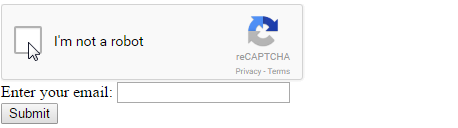When the client checks the "I'm not a robot" box, a visual selection menu appears over the top of the form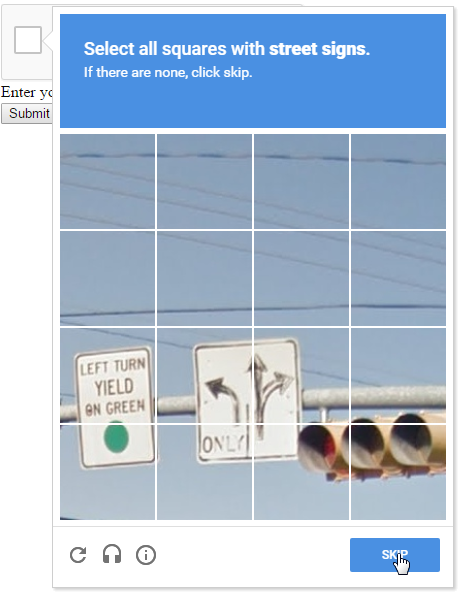Once the client has followed the instructions, a "verify" button appearsUpon clicking the "verify" button, Google will assess the client's ability to follow the visual instructions.  This process may be repeated for one or two more image tests, but it's generally easy and unobtrusive.  When the client completes the visual exercises, Google injects g-recaptcha-response into the form, removes the visual overlay, and allows the form to be completed and submitted.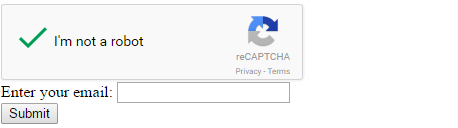In the action script, you will find the g-recaptcha-response element in \$_POST.  Following the guidance in the code snippet above, you POST this response back to Google and Google returns a JSON string telling you if the client successfully passed the visual tests.  If the "success" value is not "true" you can discard the request.

You can use PHP to create an image-based CAPTCHA test of your own.  Our image-based CAPTCHA test will use a similar strategy -- to ask the client to enter a random 5-character string of letters and numbers.  To begin, we need a function to generate the character string.  This code snippet will do the job.

```<?php // RAY_EE_captcha_sanity.php
error_reporting(E_ALL);

// FUNCTION TO MAKE A RANDOM STRING
function random_string(\$length=5)
{
// POSSIBLE PERMUATIONS http://www.mathwords.com/p/permutation_formula.htm
// pow(\$length,strlen(\$alpha)); = 32*31*30*29*28 = 24,165,120 IF LENGTH IS 5
//        1...5...10...15...20...25...30......
\$alpha = "ABCDEFGHJKLMNPQRSTUVWXYZ23456789";
\$array = str_split(\$alpha);
\$randy = NULL;
while(strlen(\$randy) < \$length)
{
// CHOOSE A LETTER AT RANDOM, USE IT AND REMOVE IT
\$point  = mt_rand(0, count(\$array)-1);
\$randy .= \$array[\$point];
unset(\$array[\$point]);
\$array = array_values(\$array);
}
return \$randy;
}

// TEST THE FUNCTION
echo random_string();```

A couple of notes about the random_string() function.  No character can be used more than once because we are planning to use the characters as array keys in our script that generates the images.  Associative arrays can only have one value per key.  That is why we remove each character as we choose it, and reset the array before choosing the next character.  By doing this we ensure that the five characters are unique.

The length of the \$alpha string has a great effect on the number of possible combinations.  Making a longer string would be easy to do - just add the lower case characters and the special characters.  But the alphabet we have already generates millions of different strings, and that seems like enough to me.  If you're willing to live with only 863,040 combinations you can make the string four characters!  The string length represents a trade-off between security and convenience.

You can also see that we intentionally left some characters out of our alphabet including O (oh), 0 (zero), I (eye) and 1 (one) because these characters can be visually confusing.  If lower case letters are added to the mix it makes sense to omit l (ell) in addition to the others.  Depending on the font you choose for the CAPTCHA string, it may make sense to omit 2 (two) and Z (zee) as well as S (ess) and 5 (five) and possibly 8 (eight).

We also need a script that can generate an image of the random five-character string.  We use an image instead of the actual characters because automation can readily detect the letters and numbers, and copy them into the form.  This would defeat the effort to tell humans and computers apart.  While some OCR programs can recognize the characters inside an image, they can often be confounded if we distort the image a little bit.  We can use the PHP image functions to read in standard images of the characters and apply some distortion.  In PHP the image functions are available if PHP is compiled with the bundled version of the GD library.  Most modern PHP installations will include the GD library.

Some Things That Did Not Work Very Well

I experimented with letting PHP generate the text, using ImageString(). The first CAPTCHA images looked something like this, and I felt that the images might be too easy to decode.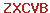Next I tried adding some colorization and image distortion.  It worked, but even the largest letters that ImageString() created seemed too small to me, and some of the rotations made some of the letters visually confusing.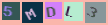As a person of limited vision (in many senses of the term) I wanted larger letters, so I created my own alphabet images using the Georgia font.  I made two-color black and white images of each letter.  The attached PSD file (with layers for each letter) was my original file.  Using Photoshop I saved each letter individually into its own PNG.  Taken together, the entire collection of PNG images is less than 100KB.  They look like this.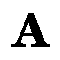Creating an Automated Test Script

Working with PHP image manipulation functions is complicated stuff, and it stands to reason that we will need to do a lot of testing.  Anything we can do that makes the testing faster and easier makes sense.  The first script we want to write is the test script that creates a CAPTCHA-protected form.  To do that, our script generates the CAPTCHA string and stores it in the \$_SESSION array.  Then it creates the HTML form.  In this script we combine the form script and the action script into one script file.   The action script is the first part of this script file.

There are three essential elements to testing the CAPTCHA response from the client.  First, was the form posted (line 10)? Second, is there a CAPTCHA string in the session array (line 13)? Third, does the client input match the CAPTCHA string (line 16)?  If these three tests are satisfied, we can process the form input (line 19).  If either the second or third test fail, we do not process the form input and instead issue a message to the client (lines 24 or 29).

If the form was not posted at all, we just skip the action part of this script and instead run the part that generates the CAPTCHA string and calls the image generator (lines 35-47).

As you read this script over, note the use of strtoupper().  This has the effect of normalizing the client input to upper case and normalizing the CAPTCHA string to upper case.  In other words, the entire process is made case-insensitive.  The test script looks like this.

```<?php // RAY_EE_captcha_sanity_in_action.php
error_reporting(E_ALL);

// REQUIRED FOR CARRYING THE RANDOM CAPTCHA STRING
session_start();

// IF ANYTHING WAS POSTED
if (!empty(\$_POST))
{
// IF THERE IS A CAPTCHA STRING IN THE SESSION ARRAY
{
// IF THE STRINGS MATCH (CASE-INSENSITIVE)
{
// WE CAN NOW PROCESS THE FORM INPUT
echo "SUCCESS!";
}
else
{
// MIGHT WANT TO MAKE THIS USER-FRIENDLY
echo 'SECURITY CODE NUMBER DID NOT MATCH';
}
}
else
{
echo 'SECURITY CODE NUMBER IS MISSING - MAYBE THE SESSION TIMED OUT?';
}
}
// END OF PHP ACTION SCRIPT - PUT UP THE FORM

// STORE THE RANDOM STRING IN THE SESSION FOR USE BY THE IMAGE GENERATOR

\$form = <<<FORM
<form method="post">
Type the security code you see below:
<input name="typed" type="text" autocomplete="off" />
<input type="submit" />
</form>
FORM;

echo \$form;

// FUNCTION TO MAKE A RANDOM STRING
function random_string(\$length=5)
{
// POSSIBLE PERMUATIONS http://www.mathwords.com/p/permutation_formula.htm
// pow(\$length,strlen(\$alpha)); = 32*31*30*29*28 = 24,165,120 IF LENGTH IS 5
//        1...5...10...15...20...25...30......
\$alpha = "ABCDEFGHJKLMNPQRSTUVWXYZ23456789";
\$array = str_split(\$alpha);
\$randy = NULL;
while(strlen(\$randy) < \$length)
{
// CHOOSE A LETTER AT RANDOM, USE IT AND REMOVE IT
\$point  = mt_rand(0, count(\$array)-1);
\$randy .= \$array[\$point];
unset(\$array[\$point]);
\$array = array_values(\$array);
}
return \$randy;
}```

Turning a Character String into an Image

Now we are ready to begin developing the CAPTCHA image generator using the alphabet of PNG images.  The strategy is to create images of letters and numbers that are rotated and distorted in ways that would be able to confuse an OCR program, but would still be readable to humans.

Image manipulation in PHP requires some depth of understanding about the various image functions and they can be complicated things to remember, so to make an easy reference we put the man page URLs into the script comments near the top at lines 5-17.

Script initialization occurs at the top in lines 20-35.  Since we are working with predefined image files, we need to know their size and the URL path, and we need to know what alphanumeric characters to put into the image.

Beginning on line 39 we process each character one at a time.  You can choose whether you like colorful letters or black and white letters.  That choice is made in the \$rgb array assigned in lines 41-54.  You can omit one of these definitions.

We select the foreground and background color at random (lines 57-59).  We can do this because the colors in the \$rgb array are of strong contrasts.

We load the alphanumeric image into a PHP image resource (line 62) and assign our chosen foreground and background colors to the image (lines 64-82).  This algorithm merits some discussion.

Assigning Colors to Paletted Images

You may be familiar with the RGB color notation.  The values for red, green or blue range from zero for no output to 255 for maximum output.  These values give the amount of on-screen brightness for each of these three color elements.  In round numbers, there are 16 million possible colors in the RGB "spectrum" and that is usually far more than the actual number of colors in any given image.  Paletted images achieve a relatively small size by keeping a numeric record of which colors are actually present in the image, and associating the RGB values with a "color index" for each pixel.

We know that we are working with a paletted two-color image because we created the PNG files that represent the characters in our alphabet.  But we do not necessarily know what the palette positions or values are.  So we make a diagonal pass across the image, starting in the upper left-hand corner and ending in the lower right-hand corner (lines 64-72).  By sampling the pixels this way we know that the first pixel we find at position 0,0 will be the background color, and the only other color we detect will be the foreground color.  For each pixel we sample, we assign the color index to \$dot using the PHP function ImageColorAt().  Then we place the color index into the \$colors array using the color index as both the array key and the array value.  This causes identical color indexes to overwrite each other.  At the end of this loop we have an array giving all of the unique color indexes in the image, which in our case is only two colors.

In PHP, a good rule of thumb is that you always assign the background color first, and we do that using our randomly chosen colors (line 78).  We also save this background color for later use when we twist the letters.  Then we assign the foreground color and the process of semi-random image colorization is complete for this character.

Rotating and Distorting the Character Images

Next we want to twist the character, so we choose a random amount of rotation and a random direction (lines 85-89).  Then we apply the ImageRotate() function.  This process creates a new image resource that will be bigger than the original image and we will have to deal with that later.

At this point we can apply ImageFilter() effects to the twisted letter.  You might experiment with these - it is more art than science.  I found that the IMAGE_FILTER_EMBOSS produced an effect that looked good.  I also left some of the other code in this script in the form of comments at lines 103-106.  You might try activating these lines to see how the other image filters work.

Copying, Resizing and Recentering a Rotated Image

Next we need to recenter the image in the 60x60 pixel size, but since we have used random rotations, we do not know what size the rotated image has assumed.  However we do know that the part of the rotated image we want to keep is in the middle.  So we can take the new larger dimensions and subtract the original smaller \$size and divide by 2 (lines 109-110).  This will give us both the X and Y offset into the rotated image. When we copy from this offset and keep \$size pixels in each dimension we will get the part of the image we want to keep and we will discard the excess background area.

We create a new image resource, reusing the character's position in the \$images array (line 111), assign a background color (line 112) and copy the center of the twisted letter into the image resource (line 114-124).

This process is repeated for each of the letters or numbers in the CAPTCHA string.  At the end of this loop we have an array of twisted and distorted images of the characters.  We need to copy the characters into a single image for browser display.

Bringing the Character Images Together for Browser Display

Getting the final image output size is easy - it is the number of letters multiplied by the width (line 128).  Because we are going to do some later processing with transparency, we use ImageSaveAlpha() to set the appropriate flags in the \$out image resource (line 129).  Then we copy each of the letters from the \$images array into the \$out image.

Working with Transparent Pixels

The next part of the process is entirely optional - again a question more of art than science - and I left the code in this script to show how to handle some of the issues related to image transparency.  It is not entirely intuitive.

Our goal here is to speckle the image with transparency.  This will allow a contrasting background color to show through the CAPTCHA image when it is rendered by the browser.  To do that we must tell the image resource what color will be considered transparent.  First, we shut off alpha blending (line 150) then choose the color we will make transparent and the degree of transparency (line 151).  The transparency value of 127 is 100% transparent.  The speckling process is done one pixel at a time (line 160) in a semi-random method by the code in lines 152-165.  Finally we turn the alpha blending back on (line 166) and the pixels we filled with the value of \$speck are made transparent.  Our image is complete.

The last step is to write the image into the browser output stream (line 168, et seq).

```<?php // RAY_EE_captcha_sanity_image.php
error_reporting(E_ALL);

// GENERATES A PICTURE OF A CHARACTER STRING INTO THE BROWSER OUTPUT
// MAN PAGE http://php.net/manual/en/function.imagecreatefrompng.php
// MAN PAGE http://php.net/manual/en/function.imagecolorat.php
// MAN PAGE http://php.net/manual/en/function.imagecolorset.php
// MAN PAGE http://php.net/manual/en/function.imagerotate.php
// MAN PAGE http://php.net/manual/en/function.imagefilter.php
// MAN PAGE http://php.net/manual/en/function.imagesx.php
// MAN PAGE http://php.net/manual/en/function.imagecreatetruecolor.php
// MAN PAGE http://php.net/manual/en/function.imagecopy.php
// MAN PAGE http://php.net/manual/en/function.imagesavealpha.php
// MAN PAGE http://php.net/manual/en/function.imagealphablending.php
// MAN PAGE http://php.net/manual/en/function.imagecolorallocatealpha.php
// MAN PAGE http://php.net/manual/en/function.imagepng.php

// REQUIRED FOR CARRYING THE CAPTCHA STRING
session_start();

// SOURCE IMAGES MUST BE SQUARE, CENTERED AND THIS SIZE
\$size = 60;

// PATH TO THE COLLECTION OF LETTER IMAGES

if (!\$letters) die('UNABLE TO LOCATE THE CAPTCHA STRING IN THE SESSION ARRAY');

// ARRAY POSITIONS FOR EACH LETTER IN THE CAPTCHA TEXT
\$images   = array();
\$bgcolors = array();

// PROCESS EACH LETTER ONE AT A TIME
\$letters  = str_split(\$letters);
foreach (\$letters as \$ltr)
{
// CHOOSE COLORFUL LETTERS
\$rgb = array
( array( 'r' => 255, 'g' =>   0, 'b' =>   0 ) // RED
, array( 'r' =>   0, 'g' => 255, 'b' =>   0 ) // GREEN
, array( 'r' =>   0, 'g' =>   0, 'b' => 255 ) // BLUE
)
;

// CHOOSE GRAY-SCALE LETTERS
\$rgb = array
( array( 'r' =>   0, 'g' =>   0, 'b' =>   0 ) // BLACK
, array( 'r' => 255, 'g' => 255, 'b' => 255 ) // WHITE
)
;

// MAKE A RANDOM SELECTION OF BACKGROUND AND FOREGROUND
shuffle(\$rgb);
\$bgc = array_pop(\$rgb);
\$fgc = array_pop(\$rgb);

// CREATE THE IMAGE RESOURCE FOR THIS LETTER
\$images[\$ltr] = imageCreateFromPNG(\$path . \$ltr . '.png');

// GET THE PALETTE INDEXES FOR THE TWO COLOR IMAGE
\$pixel  = 0;
\$colors = array();
while (\$pixel < \$size)
{
\$dot = imageColorAt(\$images[\$ltr], \$pixel, \$pixel);
\$colors[\$dot] = \$dot;
\$pixel++;
}

// POSITION ZERO IS BG, POSITION ONE IS THE LETTER
\$colors = array_values(\$colors);

// ASSIGN THE BACKGROUND COLOR
imageColorSet(\$images[\$ltr], \$colors, \$bgc['r'], \$bgc['g'], \$bgc['b']);
\$bgcolors[\$ltr] = \$colors;

// ASSIGN THE FOREGROUND COLOR
imageColorSet(\$images[\$ltr], \$colors, \$fgc['r'], \$fgc['g'], \$fgc['b']);

// CREATE RANDOM ROTATION VALUES (DEGREES ARE ANTI-CLOCKWISE)
\$twist = rand(15,40);
if (rand(0,1))
{
\$twist  = 360 - \$twist;
}

// ROTATE THE IMAGE - CREATES NEW IMAGE RESOURCES
\$twisted[\$ltr]
= imageRotate
( \$images[\$ltr]   // STARTING IMAGE RESOURCE
, \$twist          // ROTATION ANGLE
, \$bgcolors[\$ltr] // BACKGROUND COLOR
)
;

// DAMAGE THE IMAGE A LITTLE BIT TO HAMPER THE OCR-BOTS
imageFilter(\$twisted[\$ltr], IMG_FILTER_EMBOSS);

// FOR A WHITE BACKGROUND, USE GRAY-SCALE LETTERS AND ACTIVATE THESE LINES
// imageFilter(\$twisted[\$ltr], IMG_FILTER_MEAN_REMOVAL);
// imageFilter(\$twisted[\$ltr], IMG_FILTER_BRIGHTNESS, 128);
// imageFilter(\$twisted[\$ltr], IMG_FILTER_CONTRAST, -128);

// GET THE NEW DIMENSIONS AFTER ROTATION AND RECENTER THE IMAGE
\$imagesx = imagesX(\$twisted[\$ltr]) - \$size;
\$imagesx = floor(\$imagesx / 2);
\$images[\$ltr] = imageCreateTrueColor(\$size, \$size);
imageColorSet(\$images[\$ltr], \$colors, \$bgc['r'], \$bgc['g'], \$bgc['b']);

imageCopy
( \$images[\$ltr]  // DESTINATION IMAGE RESOURCE
, \$twisted[\$ltr] // SOURCE IMAGE RESOURCE
, 0              // DESTINATION X-COORDINATE
, 0              // DESTINATION Y-COORDINATE
, \$imagesx       // SOURCE X-COORDINATE
, \$imagesx       // SOURCE Y-COORDINATE
, \$size          // WIDTH OF SOURCE
, \$size          // HEIGHT OF SOURCE
)
;
}

// COMPUTE THE SIZE OF THE FINAL OUTPUT IMAGE
\$out = imageCreateTrueColor( (count(\$letters) * \$size), \$size);
imageSaveAlpha(\$out, TRUE);

// COPY THE LETTERS INTO THE OUTPUT IMAGE
\$x = 0;
foreach (\$images as \$ltr => \$img)
{
imageCopy
( \$out            // DESTINATION IMAGE RESOURCE
, \$img            // SOURCE IMAGE RESOURCE
, \$x              // DESTINATION X-COORDINATE
, 0               // DESTINATION Y-COORDINATE
, 0               // SOURCE X-COORDINATE
, 0               // SOURCE Y-COORDINATE
, \$size           // WIDTH OF SOURCE
, \$size           // HEIGHT OF SOURCE
)
;
\$x += \$size;
}

// SPECKLE THE IMAGE WITH TRANSPARENCY
imageAlphaBlending(\$out, FALSE);
\$speck = imageColorAllocateAlpha(\$out, 255, 255, 255, 127);
\$x = imagesX(\$out)-1;
\$y = imagesY(\$out)-1;
\$w = rand(0,1);
\$h = rand(0,1);
while (\$w < \$x)
{
while (\$h < \$y)
{
imageSetPixel(\$out, \$w, \$h, \$speck);
\$h += rand(2,4);
}
\$w += 2;
\$h = rand(0,1);
}
imageAlphaBlending(\$out, TRUE);

// SEND THE IMAGE INTO THE BROWSER OUTPUT STREAM
imagePNG(\$out);
imageDestroy(\$out);```

How Does It Look?

Here is what our new CAPTCHA images will look like, depending on whether we chose the color or the grayscale values in the \$rgb array.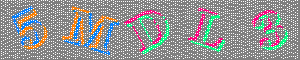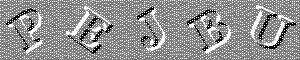A Word About Accessibility

For clients of poor vision or those using screen readers, CAPTCHA tests can pose real problems.  A way around this issue is to create an audio CAPTCHA.  Each letter and number of the \$alpha array can be made to correspond to an audio file telling the character in an unambiguous way.  The audio CAPTCHA script could play each of the audio files.  These files would use the radio alphabet to disambiguate similar-sounding letters and numbers.  As an example, the audio version of our colorful CAPTCHA image might say this: Five, M as in Mike, D as in Delta, L as in Lima, Three.

The FAA approved radio alphabet uses the following expressions.

```\$alphabet_array["A"] = "Alfa";
\$alphabet_array["B"] = "Bravo";
\$alphabet_array["C"] = "Charlie";
\$alphabet_array["D"] = "Delta";
\$alphabet_array["E"] = "Echo";
\$alphabet_array["F"] = "Foxtrot";
\$alphabet_array["G"] = "Golf";
\$alphabet_array["H"] = "Hotel";
\$alphabet_array["I"] = "India";
\$alphabet_array["J"] = "Juliette";
\$alphabet_array["K"] = "Kilo";
\$alphabet_array["L"] = "Lima";
\$alphabet_array["M"] = "Mike";
\$alphabet_array["N"] = "November";
\$alphabet_array["O"] = "Oskar";
\$alphabet_array["P"] = "Papa";
\$alphabet_array["Q"] = "Quebec";
\$alphabet_array["R"] = "Romeo";
\$alphabet_array["S"] = "Sierra";
\$alphabet_array["T"] = "Tango";
\$alphabet_array["U"] = "Uniform";
\$alphabet_array["V"] = "Viktor";
\$alphabet_array["W"] = "Whiskey";
\$alphabet_array["X"] = "Xray";
\$alphabet_array["Y"] = "Yankee";
\$alphabet_array["Z"] = "Zulu";

\$alphabet_array["0"] = "Zero";
\$alphabet_array["1"] = "One";
\$alphabet_array["2"] = "Two";
\$alphabet_array["3"] = "Three";
\$alphabet_array["4"] = "Four";
\$alphabet_array["5"] = "Five";
\$alphabet_array["6"] = "Six";
\$alphabet_array["7"] = "Seven";
\$alphabet_array["8"] = "Eight";
\$alphabet_array["9"] = "Niner";```

Summary

With a little bit of programming and 32 stored PNG files we have freed ourselves from dependency on a foreign web service and given our clients a secure form that is easier on the eyes.  These scripts can be installed on your server and used as-is to duplicate what you see here.  The attached PSD file contains one layer for each of the letters.  You can open it in Photoshop or similar drawing programs, render each layer visible one at a time, and save the file as a PNG, named in such a way that each image file matches a character in our alphabet string.  Be sure to coordinate the file path with the expected \$path in the image generator script (line 27).

The PSD File with the Letters in Layers

Just for Fun - the Worst Captcha Ever